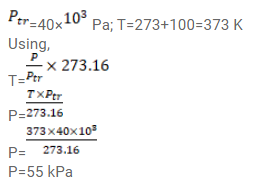# The pressure measured by a constant volume gas thermometer isQuestion:

The pressure measured by a constant volume gas thermometer is $40 \mathrm{kPa}$ at the triple point of water. What will be the pressure measured at the boiling point of water $\left(100^{\circ} \mathrm{C}\right)$ ?

Solution: